Publicité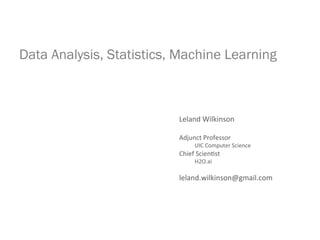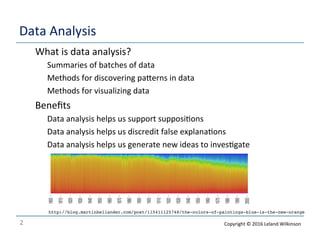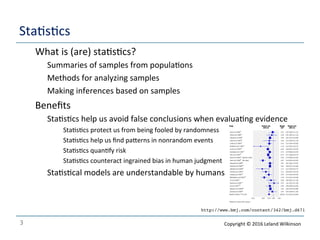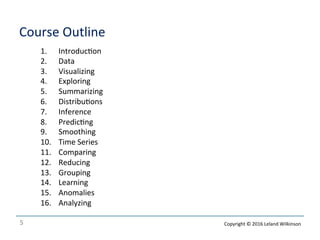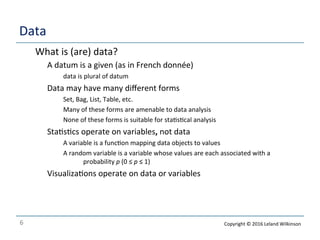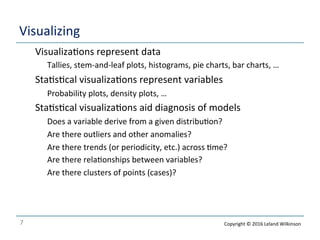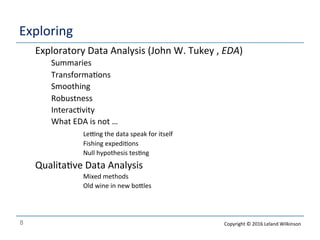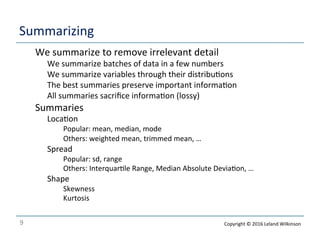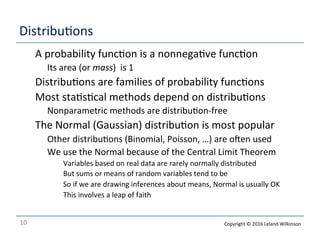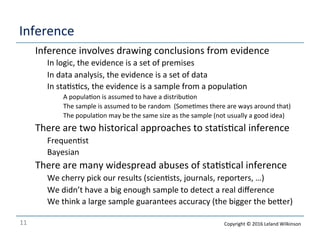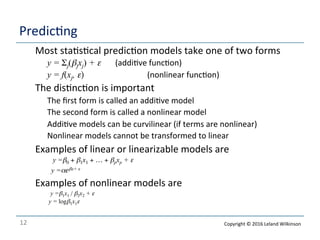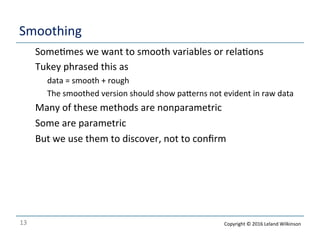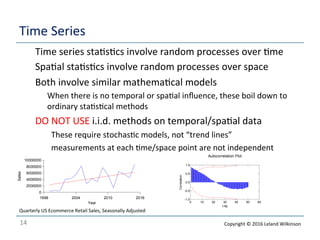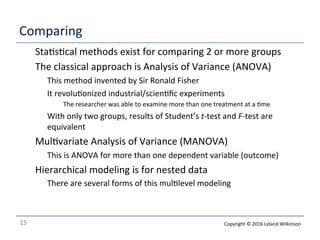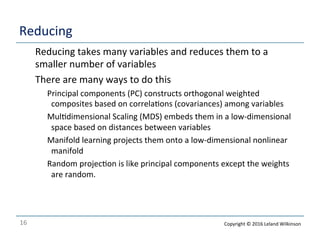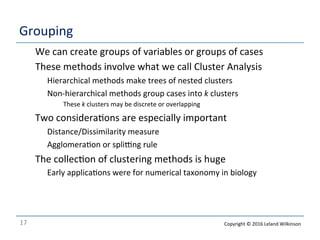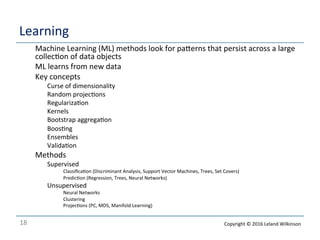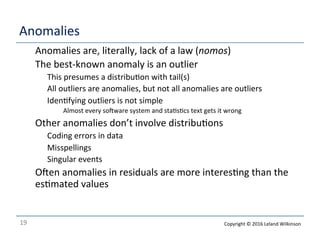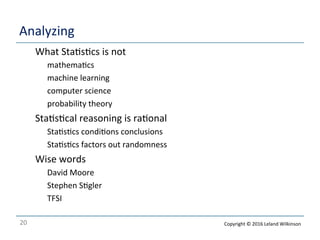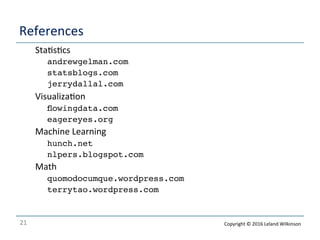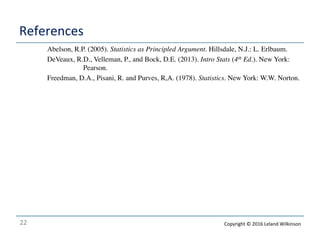1 sur 22
Publicité

### Session 01 (Introduction).pdf

1. Data Analysis, Statistics, Machine Learning Leland  Wilkinson     Adjunct  Professor                        UIC  Computer  Science   Chief  Scien<st                        H2O.ai     leland.wilkinson@gmail.com
2. 2   Data  Analysis   o What  is  data  analysis?   o Summaries  of  batches  of  data   o Methods  for  discovering  paJerns  in  data   o Methods  for  visualizing  data   o Beneﬁts   o Data  analysis  helps  us  support  supposi<ons   o Data  analysis  helps  us  discredit  false  explana<ons   o Data  analysis  helps  us  generate  new  ideas  to  inves<gate   http://blog.martinbellander.com/post/115411125748/the-colors-of-paintings-blue-is-the-new-orange Copyright  ©  2016  Leland  Wilkinson
3. 3   Sta<s<cs   o What  is  (are)  sta<s<cs?   o Summaries  of  samples  from  popula<ons   o Methods  for  analyzing  samples     o Making  inferences  based  on  samples     o Beneﬁts   o Sta<s<cs  help  us  avoid  false  conclusions  when  evalua<ng  evidence   o Sta<s<cs  protect  us  from  being  fooled  by  randomness   o Sta<s<cs  help  us  ﬁnd  paJerns  in  nonrandom  events   o Sta<s<cs  quan<fy  risk   o Sta<s<cs  counteract  ingrained  bias  in  human  judgment   o Sta<s<cal  models  are  understandable  by  humans   http://www.bmj.com/content/342/bmj.d671 Copyright  ©  2016  Leland  Wilkinson
4. 4   Machine  Learning   o What  is  machine  learning?   o Data  mining  systems     o Discover  paJerns  in  data   o Learning  systems     o Adapt  models  over  <me   o Beneﬁts   o ML  helps  to  predict  outcomes   o ML  oXen  outperforms  tradi<onal  sta<s<cal  predic<on  methods   o ML  models  do  not  need  to  be  understood  by  humans   o Most  ML  results  are  unintelligible  (the  excep<ons  prove  the  rule)   o ML  people  care  about  the  quality  of  a  predic<on,  not  the  meaning  of  the  result   o ML  is  hot  (Deep  Learning!,  Big  Data!)   http://swift.cmbi.ru.nl/teach/B2/bioinf_24.html Copyright  ©  2016  Leland  Wilkinson
5. 5   Course  Outline   1. Introduc<on   2. Data   3. Visualizing   4. Exploring   5. Summarizing   6. Distribu<ons   7. Inference   8. Predic<ng   9. Smoothing   10. Time  Series   11. Comparing   12. Reducing   13. Grouping   14. Learning   15. Anomalies   16. Analyzing   Copyright  ©  2016  Leland  Wilkinson
6. 6   Data   o What  is  (are)  data?   o A  datum  is  a  given  (as  in  French  donnée)   o data  is  plural  of  datum   o Data  may  have  many  diﬀerent  forms   o Set,  Bag,  List,  Table,  etc.   o Many  of  these  forms  are  amenable  to  data  analysis   o None  of  these  forms  is  suitable  for  sta<s<cal  analysis   o Sta<s<cs  operate  on  variables,  not  data   o A  variable  is  a  func<on  mapping  data  objects  to  values   o A  random  variable  is  a  variable  whose  values  are  each  associated  with  a    probability  p  (0  ≤  p  ≤  1)   o Visualiza<ons  operate  on  data  or  variables   Copyright  ©  2016  Leland  Wilkinson
7. 7   Visualizing   o Visualiza<ons  represent  data   o Tallies,  stem-­‐and-­‐leaf  plots,  histograms,  pie  charts,  bar  charts,  …   o Sta<s<cal  visualiza<ons  represent  variables   o Probability  plots,  density  plots,  …   o Sta<s<cal  visualiza<ons  aid  diagnosis  of  models   o Does  a  variable  derive  from  a  given  distribu<on?   o Are  there  outliers  and  other  anomalies?   o Are  there  trends  (or  periodicity,  etc.)  across  <me?   o Are  there  rela<onships  between  variables?   o Are  there  clusters  of  points  (cases)?   Copyright  ©  2016  Leland  Wilkinson
8. 8   Exploring   o Exploratory  Data  Analysis  (John  W.  Tukey  ,  EDA)    Summaries    Transforma<ons    Smoothing    Robustness    Interac<vity    What  EDA  is  not  …      Lelng  the  data  speak  for  itself      Fishing  expedi<ons      Null  hypothesis  tes<ng   Qualita<ve  Data  Analysis      Mixed  methods      Old  wine  in  new  boJles   Copyright  ©  2016  Leland  Wilkinson
9. 9   Summarizing   o We  summarize  to  remove  irrelevant  detail   o We  summarize  batches  of  data  in  a  few  numbers   o We  summarize  variables  through  their  distribu<ons   o The  best  summaries  preserve  important  informa<on   o All  summaries  sacriﬁce  informa<on  (lossy)   o Summaries   o Loca<on   o Popular:  mean,  median,  mode   o Others:  weighted  mean,  trimmed  mean,  …   o Spread   o Popular:  sd,  range   o Others:  Interquar<le  Range,  Median  Absolute  Devia<on,  …   o Shape   o Skewness   o Kurtosis     Copyright  ©  2016  Leland  Wilkinson
10. 10   Distribu<ons   o A  probability  func<on  is  a  nonnega<ve  func<on   o Its  area  (or  mass)    is  1   o Distribu<ons  are  families  of  probability  func<ons   o Most  sta<s<cal  methods  depend  on  distribu<ons   o Nonparametric  methods  are  distribu<on-­‐free   o The  Normal  (Gaussian)  distribu<on  is  most  popular   o Other  distribu<ons  (Binomial,  Poisson,  …)  are  oXen  used   o We  use  the  Normal  because  of  the  Central  Limit  Theorem   o Variables  based  on  real  data  are  rarely  normally  distributed   o But  sums  or  means  of  random  variables  tend  to  be   o So  if  we  are  drawing  inferences  about  means,  Normal  is  usually  OK   o This  involves  a  leap  of  faith     Copyright  ©  2016  Leland  Wilkinson
11. 11   Inference   o Inference  involves  drawing  conclusions  from  evidence   o In  logic,  the  evidence  is  a  set  of  premises   o In  data  analysis,  the  evidence  is  a  set  of  data   o In  sta<s<cs,  the  evidence  is  a  sample  from  a  popula<on   o A  popula<on  is  assumed  to  have  a  distribu<on   o The  sample  is  assumed  to  be  random    (Some<mes  there  are  ways  around  that)   o The  popula<on  may  be  the  same  size  as  the  sample  (not  usually  a  good  idea)   o There  are  two  historical  approaches  to  sta<s<cal  inference   o Frequen<st   o Bayesian   o There  are  many  widespread  abuses  of  sta<s<cal  inference   o We  cherry  pick  our  results  (scien<sts,  journals,  reporters,  …)   o We  didn’t  have  a  big  enough  sample  to  detect  a  real  diﬀerence   o We  think  a  large  sample  guarantees  accuracy  (the  bigger  the  beJer)     Copyright  ©  2016  Leland  Wilkinson
12. 12   Predic<ng   o Most  sta<s<cal  predic<on  models  take  one  of  two  forms     o y = Σj(βjxj) + ε (addi<ve  func<on) o y = f(xj, ε) (nonlinear  func<on) o The  dis<nc<on  is  important   o The  ﬁrst  form  is  called  an  addi<ve  model   o The  second  form  is  called  a  nonlinear  model   o Addi<ve  models  can  be  curvilinear  (if  terms  are  nonlinear)   o Nonlinear  models  cannot  be  transformed  to  linear     o Examples  of  linear  or  linearizable  models  are   o  y =β0 + β1x1 + … + βpxp + ε o y =αeβx+ ε o Examples  of  nonlinear  models  are   o  y =β1x1 / β2x2 + ε o  y = logβ1x1ε   Copyright  ©  2016  Leland  Wilkinson
13. 13   Smoothing   o Some<mes  we  want  to  smooth  variables  or  rela<ons   o Tukey  phrased  this  as   o data  =  smooth  +  rough   o The  smoothed  version  should  show  paJerns  not  evident  in  raw  data   o Many  of  these  methods  are  nonparametric   o Some  are  parametric   o But  we  use  them  to  discover,  not  to  conﬁrm       Copyright  ©  2016  Leland  Wilkinson
14. 14   Time  Series   o Time  series  sta<s<cs  involve  random  processes  over  <me   o Spa<al  sta<s<cs  involve  random  processes  over  space   o Both  involve  similar  mathema<cal  models   o When  there  is  no  temporal  or  spa<al  inﬂuence,  these  boil  down  to   ordinary  sta<s<cal  methods   DO  NOT  USE  i.i.d.  methods  on  temporal/spa<al  data    These  require  stochas<c  models,  not  “trend  lines”    measurements  at  each  <me/space  point  are  not  independent       1998 2004 2010 2016 Year 0 2000000 4000000 6000000 8000000 10000000 Sales Autocorrelation Plot 0 10 20 30 40 50 60 Lag -1.0 -0.5 0.0 0.5 1.0 Correlation Quarterly  US  Ecommerce  Retail  Sales,  Seasonally  Adjusted   Copyright  ©  2016  Leland  Wilkinson
15. 15   Comparing   o Sta<s<cal  methods  exist  for  comparing  2  or  more  groups   o The  classical  approach  is  Analysis  of  Variance  (ANOVA)   o This  method  invented  by  Sir  Ronald  Fisher   o It  revolu<onized  industrial/scien<ﬁc  experiments   o The  researcher  was  able  to  examine  more  than  one  treatment  at  a  <me   o With  only  two  groups,  results  of  Student’s  t-­‐test  and  F-­‐test  are   equivalent   o Mul<variate  Analysis  of  Variance  (MANOVA)   o This  is  ANOVA  for  more  than  one  dependent  variable  (outcome)   o Hierarchical  modeling  is  for  nested  data   o There  are  several  forms  of  this  mul<level  modeling       Copyright  ©  2016  Leland  Wilkinson
16. 16   Reducing   o Reducing  takes  many  variables  and  reduces  them  to  a   smaller  number  of  variables   o There  are  many  ways  to  do  this   o Principal  components  (PC)  constructs  orthogonal  weighted      composites  based  on  correla<ons  (covariances)  among  variables   o Mul<dimensional  Scaling  (MDS)  embeds  them  in  a  low-­‐dimensional    space  based  on  distances  between  variables   o Manifold  learning  projects  them  onto  a  low-­‐dimensional  nonlinear    manifold   o Random  projec<on  is  like  principal  components  except  the  weights    are  random.   Copyright  ©  2016  Leland  Wilkinson
17. 17   Grouping   o We  can  create  groups  of  variables  or  groups  of  cases   o These  methods  involve  what  we  call  Cluster  Analysis   o Hierarchical  methods  make  trees  of  nested  clusters   o Non-­‐hierarchical  methods  group  cases  into  k  clusters   o These  k  clusters  may  be  discrete  or  overlapping   o Two  considera<ons  are  especially  important   o Distance/Dissimilarity  measure   o Agglomera<on  or  splilng  rule   o The  collec<on  of  clustering  methods  is  huge   o Early  applica<ons  were  for  numerical  taxonomy  in  biology         Copyright  ©  2016  Leland  Wilkinson
18. 18   Learning   o Machine  Learning  (ML)  methods  look  for  paJerns  that  persist  across  a  large   collec<on  of  data  objects   o ML  learns  from  new  data   o Key  concepts   o Curse  of  dimensionality   o Random  projec<ons   o Regulariza<on   o Kernels   o Bootstrap  aggrega<on   o Boos<ng   o Ensembles   o Valida<on   o Methods   o Supervised   o Classiﬁca<on  (Discriminant  Analysis,  Support  Vector  Machines,  Trees,  Set  Covers)   o Predic<on  (Regression,  Trees,  Neural  Networks)   o Unsupervised   o Neural  Networks   o Clustering   o Projec<ons  (PC,  MDS,  Manifold  Learning)       Copyright  ©  2016  Leland  Wilkinson
19. 19   Anomalies   o Anomalies  are,  literally,  lack  of  a  law  (nomos)   o The  best-­‐known  anomaly  is  an  outlier   o This  presumes  a  distribu<on  with  tail(s)   o All  outliers  are  anomalies,  but  not  all  anomalies  are  outliers   o Iden<fying  outliers  is  not  simple   o Almost  every  soXware  system  and  sta<s<cs  text  gets  it  wrong   o Other  anomalies  don’t  involve  distribu<ons   o Coding  errors  in  data   o Misspellings   o Singular  events   o OXen  anomalies  in  residuals  are  more  interes<ng  than  the   es<mated  values       Copyright  ©  2016  Leland  Wilkinson
20. 20   Analyzing   o What  Sta<s<cs  is  not     o mathema<cs   o machine  learning   o computer  science   o probability  theory   o Sta<s<cal  reasoning  is  ra<onal   o Sta<s<cs  condi<ons  conclusions   o Sta<s<cs  factors  out  randomness   o Wise  words   o David  Moore   o Stephen  S<gler   o TFSI     Copyright  ©  2016  Leland  Wilkinson
21. 21   References   o Sta<s<cs   o andrewgelman.com o statsblogs.com o jerrydallal.com   o Visualiza<on   o flowingdata.com o eagereyes.org o Machine  Learning   o hunch.net o nlpers.blogspot.com o Math   o quomodocumque.wordpress.com o terrytao.wordpress.com Copyright  ©  2016  Leland  Wilkinson
22. 22   References   o Abelson, R.P. (2005). Statistics as Principled Argument. Hillsdale, N.J.: L. Erlbaum. o DeVeaux, R.D., Velleman, P., and Bock, D.E. (2013). Intro Stats (4th Ed.). New York: Pearson. o Freedman, D.A., Pisani, R. and Purves, R,A. (1978). Statistics. New York: W.W. Norton. Copyright  ©  2016  Leland  Wilkinson
Publicité